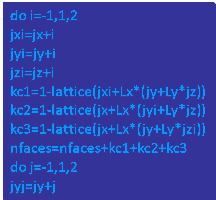Computational
Physics
Research: Algorithms## Time-dependent Schrödinger equation

Methods to solve the time-dependent Schrödinger equation and their applications are described in the following

Selected publications:
• H. De Raedt,
"Computer Simulation of Quantum Phenomena in Nano-Scale Devices",
Annual Reviews of Computational Physics IV,
ed. D. Stauffer,
World Scientific, 107 - 146 (1996)
PDF
• H. De Raedt and K. Michielsen,
"Algorithm to Solve the Time-Dependent Schrödinger Equation for a Charged Particle in an Inhomogeneous Magnetic Field: Application to the Aharonov-Bohm Eﬀect",
Comp. in Phys. 8, 600 – 607 (1994)
• H. De Raedt,
"Product Formula Algorithms for Solving the Time-Dependent Schrödinger Equation",
Comp. Phys. Rep. 7, 1 – 72 (1987)
Presentations:
• Computer animation of electron motion in nano-structures
Powerpoint

## Quantum spin dynamics

Methods to solve quantum dynamical problems that are different from the solvers of the time-dependent Schrödinger equation are described in the following

Selected publications:
• H. De Raedt and K. Michielsen,
"Computational Methods for Simulating Quantum Computers",
Handbook of Theoretical and Computational Nanotechnology, Vol. 3: Quantum and molecular computing, quantum simulations, Chapter 1, pp. 248, M. Rieth and W. Schommers eds.,American Scientific Publisher, Los Angeles (2006)
PDF
• V.V. Dobrovitski and H.A. De Raedt,
"Efficient scheme for numerical simulations of the spin-bath decoherence"
Phys. Rev. E 67, 056702 (2003)
PDF

## Time-dependent Maxwell equations

Methods to solve the time-dependent Maxwell equations are described in the following

Selected publications:
• H. De Raedt,
"Advances in Unconditionally Stable Techniques", Chapter 18 in Computational Electrodynamics: The Finite-Difference Time-Domain Method, 3rd edition,A. Taflove and S.C. Hagness, Artech House, MA USA, 2005)
• H.A. De Raedt, J.S. Kole, K.F.L. Michielsen, and M.T. Figge,
"Unified framework for numerical methods to solve the Time-Dependent Maxwell equations",
Comp. Phys. Comm. 156, 43 - 61 (2003)
PDF
• H.A. De Raedt, K.F.L. Michielsen, J.S. Kole, and M.T. Figge,
"Solving the Maxwell equations by the Chebyshev method: A one-step finite-difference time-domain algorithm",
IEEE Transactions on Antennas and Propagation 51, 3155 - 3160 (2003),
PDF
• J.S. Kole, M.T. Figge, and H. De Raedt,
"Unconditionally Stable Algorithms to Solve the Time-Dependent Maxwell Equations",
Phys. Rev. E 64, 066705 (2001)
PDF
Presentations:
• New numerical methods to solve the time-dependent Maxwell equations
Powerpoint

## Stochastic diagonalization

An alternative method (without minus-sign problem) to Quantum Monte Carlo methods to compute the smallest eigenvalue and corresponding eigenvector of very (very) large matrices is described in the following

Selected publications:
• H. De Raedt, W. Fettes, and K. Michielsen,
"Stochastic Diagonalization",
in Quantum Monte Carlo Methods in Physics and Chemistry,
eds. M.P. Nightingale and C.J. Umrigar,
NATO-ASI Series, 37 - 64 (Kluwer, The Netherlands 1999)
PDF
• K. Michielsen and H. De Raedt,
"Off-Diagonal Long-Range Order in Generalized Hubbard Models",
Int. J. Mod. Phys. B 11, 1311 - 1335 (1997)
PDF
Presentations:

## Morphological image analysis

A general method to characterize the morphology of two- and three-dimensional patterns in terms of geometrical and topological descriptors is described in the following

Selected publications:
• K. Michielsen, H. De Raedt, J.Th.M. De Hosson,
"Aspects of Mathematical Morphology"
Advances in Imaging and Electron Physics 125, 119 - 195 (2002)
PDF
• K. Michielsen and H. De Raedt,
"Integral-geometry morphological image analysis",
Phys. Rep. 347, 461 - 538 (2001)
PDF
Presentations:
• Morphological Characterization of Spatial Patterns
Powerpoint

## Event-based simulation method

The event-by-event simulation approach and its applications is described in the following

Selected publications:
• K. Michielsen, F. Jin, and H. De Raedt,
"Event-based Corpuscular Model for Quantum Optics Experiments",
J. Comp. Theor. Nanosci. 8, 1052 - 1080 (2011)
PDF
• K. De Raedt, H. De Raedt, and K. Michielsen,
"A computer program to simulate the Einstein-Podolsky-Rosen-Bohm experiments with photons",
Comp. Phys. Comm. 176, 642 - 651 (2007)
PDF
• H. De Raedt, K. De Raedt, K. Michielsen, and S. Miyashita,
"Efficient data processing and quantum phenomena: Single-particle systems"
Comp. Phys. Comm. 174, 803 - 817 (2006)
PDF
• K. De Raedt, H. De Raedt, and K. Michielsen,
" Deterministic event-based simulation of quantum phenomena",
Comp. Phys. Comm. 171, 19 - 39 (2005)
PDF
• H. De Raedt, K. De Raedt, and K. Michielsen,
"Event-based simulation of single-photon beam splitters and Mach-Zehnder interferometers",
Europhys. Lett. 69, 861 - 867 (2005)
PDF
Presentations:
• New method to simulate quantum interference using deterministic processes
Powerpoint

More information on this simulation method and its applications can be found on the website http://www.compphys.net/dlm